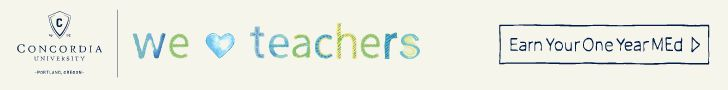# Second-Grade Mathematics StandardsIn math, your child will practice addition and subtraction skills in many different formats and learn techniques for doing math mentally. Here are a few examples of what your child will study throughout the year, questions to ask his or her teachers and tips for reinforcing math skills at home.

### Operations and Algebraic Thinking

1. Use mental math to quickly and accurately add and subtract two-digit numbers.
2. Work one- and two-step addition and subtraction problems.
Q: Jack has 10 pencils. He gives 5 pencils to a friend. He buys 3 more pencils. How many pencils does Jack have now?
A: 10 – 5 = 5 pencils
5 + 3 = 8 pencils

### Number and Operations in Base Ten

1. Understand the meaning of place value in three-digit numbers.
2. Count up to 1,000; count by fives, tens and hundreds.
5, 10, 15, 20, 25, 30, 35, …, 990, 995, 1,000
10, 20, 30, 40, 50, 60, …, 970, 980, 990, 1,000
100, 200, 300, 400, 500, 600, 700, 800, 900, 1,000

### Measurements and Data

1. Read and tell time on both digital and analog clock faces; understand the difference between a.m. and p.m.2. Measure and estimate lengths using standard and metric units.
3. Solve addition and subtraction problems using money — dollar bills, quarters, dimes, nickels, and pennies.
Q. You have 3 quarters, 10 dimes, and 2 pennies in your pocket. You find on the ground 2 more quarters. How much money do you have now?
A: 3 + 2 = 5 quarters = \$1.25; 10 dimes = \$1.00; 2 pennies = 2¢
\$1.25 + \$1.00 + \$0.02 = \$2.27
4. Do word problems that add or subtract like measurements (inches minus inches, meters plus meters).

### Geometry

1. Identify and draw common shapes, including quadrilaterals, hexagons and pentagons.
2. Divide shapes into equal parts, such as squares into cubes or circles into halves.
Q: Cut the circle in half.
A:Learn More: Click to view related resources.